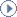Search FQXiPlease also note that we do not accept unsolicited posts and we cannot review, or open new threads for, unsolicited articles or papers. Requests to review or post such materials will not be answered. If you have your own novel physics theory or model, which you would like to post for further discussion among then FQXi community, then please add them directly to the "Alternative Models of Reality" thread, or to the "Alternative Models of Cosmology" thread. Thank you.

Forum Home
Introduction

Order posts by:
chronological order
most recent first

Display:
all posts
member posts highlighted
member posts only

RECENT POSTS IN THIS TOPIC

Steven Dinowitz: on 10/3/12 at 18:24pm UTC, wrote I have yet to see any experimental data that rules out gross violation of...

Lawrence B. Crowell: on 6/14/12 at 18:17pm UTC, wrote I thought I would outline a bit about what a dilaton is, along with the...

Brendan Foster: on 6/12/12 at 19:39pm UTC, wrote FQXi will be starting a new habit of presenting "products" from Members...

FQXi FORUM
September 26, 2021

CATEGORY: Cosmology [back]
TOPIC: Equivalence Principle Violations [refresh]

FQXi Administrator Brendan Foster wrote on Jun. 12, 2012 @ 19:39 GMT
FQXi will be starting a new habit of presenting "products" from Members supported by FQXi, here in the forums for open discussion.

To help start off this trend, please have a look at two articles from Member John Donoghue on Equivalence Principle violations using light [i.e. not heavy] fields. These articles were written as part of an FQXi-supported visit to the French physicist Thibault Damour.

Phenomenology of the Equivalence Principle with Light Scalars

Equivalence Principle Violations and Couplings of a Light Dilaton

Lawrence B. Crowell wrote on Jun. 14, 2012 @ 18:17 GMT
I thought I would outline a bit about what a dilaton is, along with the other scalar field the axion. The graviton on the closed string has two directions of oscillation, x and y. These correspond to the creation and annihilation operators

a^μ_n, (a^μ_n)^† --- > x-direction

b^μ_n, (b^μ_n)^† --- > y-direction

and we can construct a general spin or m = 1 polarization state with (a^μ_n)^† + i(b^μ_n)^†, and a m = -1 state with (a^μ_n)^† - i(b^μ_n)^†. This polarization pertains to the string parameter space. This is not the graviton state, but the composition of two of these with mode matching n and –n, due to Noether’s theorem for equal left and right moving quanta, such as

((a^μ_n)^† + i(b^μ_n)^†)((a^ν_{-n})^† + i(b^ν_{-n})^†),

gives a spin 2 field corresponding to a curvature.

The graviton is massless. This means that a spin = 2 boson can’t be placed in a reference frame where the projection of that spin onto the momentum vector is zero. In other words the graviton can’t be put in a frame where where the particle is at rest. The same holds for the photon. However, with the graviton I can compose the two polarization states into a field

((a^μ_n)^† + i(b^μ_n)^†)((a^ν_{-n})^† - i(b^ν_{-n})^†),

which as a composition of m = 1 and m = -1 polarization states means this has a spin of zero. This particle state is a scalar state and is the dilaton field. The other field with spin = 0

((a^μ_n)^† - i(b^μ_n)^†)((a^ν_{-n})^† + i(b^ν_{-n})^†),

is the axion field. The axion field is involved with the CP symmetry of the strong interaction.

It is possible to construct a spin = 1 particle state. For the entire bulk in 10 dimensions there can be other dimensions beyond μ = 0, 1, 2, 3, there are others a = 5, 6, 7, 8, 9, 10. So we can have a graviton of the form

((a^μ_n)^† + i(b^μ_n)^†)((a^5_{-n})^† + i(b^5_{-n})^†).

If we curl up that fifth dimension in a Calabi-Yau space (say a circle if we consider only this additional dimension) the closed string becomes an open string with the raising and lower operators (a^μ_n)^† + i(b^μ_n)^† and a^μ_n + ib^μ_n, and this funny operator for this internal space, which in the case of only one additional fifth dimension is a photon or a gauge vector boson

report post as inappropriate

Steven Dinowitz wrote on Oct. 3, 2012 @ 18:24 GMT
I have yet to see any experimental data that rules out gross violation of the Equivalence Principle by systems composed wholly or in part of antiquarks - in other words Antibaryons (3 antiquarks) and mesons (a quark and an antiquark).

Using the simple but revolutionary assumption that antiquarks exhibit antigravity from my essay entitled: "The Law of Conservation of Baryon Number, Antimatter Antigravity, and the Experiment that Never Gets Done" I think I have discovered a simple relationship between the mass ratio of the quark and antiquark in any given meson and the gravitational acceleration that the meson experiences. It is predicted, for example, that a neutral kaon (consisting of a d-quark and anti-s-quark) with s/d quark mass ratio of about 21 will fall up at .91g in the Earth's gravitational field. Anyone interested please see my post of 9/19/12.

report post as inappropriate